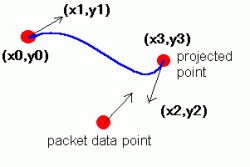# 网络游戏的移动同步（三）平滑算法

## 线性插值

 ``````1 2 3 4 5 6 7 8 `````` ``````var delta:Number = (getTimer() - rp.time) / 1000; netPlayer.targetPos = new Vector2().addVectors(rp.position, rp.velocity.clone().multiply(delta).add(rp.acceleration.clone().divide(2).multiply(delta * delta))); netPlayer.startPos = netPlayer.position.clone(); netPlayer.velocity = rp.velocity.clone(); netPlayer.acceleration = rp.acceleration.clone(); netPlayer.method = 2; netPlayer.smoothTick = netPlayer.smoothTime = delta; ``````

 `````` 1 2 3 4 5 6 7 8 9 10 11 12 13 14 15 `````` ``````if (smoothTick > 0) { smoothTick -= Global.elapse / 1000; var dt:Number = 1 - smoothTick / smoothTime; position.x = startPos.x + (targetPos.x - startPos.x) * dt; position.y = startPos.y + (targetPos.y - startPos.y) * dt; } else { if (! acceleration.isZero()) { velocity.x += acceleration.x * Global.elapse / 1000; velocity.y += acceleration.y * Global.elapse / 1000; } if (! velocity.isZero()) { position.x += velocity.x * Global.elapse / 1000; position.y += velocity.y * Global.elapse / 1000; } } ``````

Network4.swf

## 立方样条插值x = At3 + Bt2 + Ct + D

y = Et3 + Ft3 + Gt + H

A = x3 – 3x2 +3x1 – x0

B = 3x2 – 6x1 + 3x0

C = 3x1 – 3x0 D = x0

E = y3 – 3y2 +3y1 – y0

F = 3y2 – 6y1 + 3y0

G = 3y1 – 3y0

H = y0`````` 1 2 3 4 5 6 7 8 9 10 11 12 13 14 15 16 17 18 19 20 21 22 23 24 25 `````` ``````var delta:Number = (getTimer() - rp.time) / 1000; // 预测点，在延迟时间5倍以后 // 延迟越严重，预测越远 var scheduled:Number = delta * 5; scheduled = Math.min(scheduled, 0.8); var pos1:Vector2 = netPlayer.position.clone(); var pos2:Vector2 = new Vector2().addVectors(pos1, netPlayer.velocity.clone().multiply(0.1)); var pos4:Vector2 = new Vector2().addVectors(rp.position, rp.velocity.clone().multiply(scheduled).add(rp.acceleration.clone().divide(2).multiply(scheduled * scheduled))); var pos3:Vector2 = new Vector2().subVectors(pos4, rp.velocity.clone().add(rp.acceleration.clone().multiply(scheduled)).multiply(0.1)); netPlayer.smoothTick = netPlayer.smoothTime = scheduled; netPlayer.A = pos4.x - 3 * pos3.x + 3 * pos2.x - pos1.x; netPlayer.B = 3 * pos3.x - 6 * pos2.x + 3 * pos1.x; netPlayer.C = 3 * pos2.x - 3 * pos1.x; netPlayer.D = pos1.x; netPlayer.E = pos4.y - 3 * pos3.y + 3 * pos2.y - pos1.y; netPlayer.F = 3 * pos3.y - 6 * pos2.y + 3 * pos1.y; netPlayer.G = 3 * pos2.y - 3 * pos1.y; netPlayer.H = pos1.y; // 插值位置 position.x = A * dt * dt * dt + B * dt * dt + C * dt + D; position.y = E * dt * dt * dt + F * dt * dt + G * dt + H; ``````

Network4.swf

## 碰撞检测冲突

 `````` 1 2 3 4 5 6 7 8 9 10 11 12 13 14 15 16 17 18 19 20 21 22 `````` ``````// 设置修正只 netPlayer.modify.x = netPlayer.x - rp.position.x; netPlayer.modify.y = netPlayer.y - rp.position.y; //如果位置偏差实在过大，直接跳跃 if (netPlayer.modify.lengthSQ > 50 * 50) { netPlayer.modify.set(0, 0); } // 注意这里直接设置到了期望位置 netPlayer.position.x = rp.position.x; netPlayer.position.y = rp.position.y; netPlayer.velocity = rp.velocity.clone(); netPlayer.acceleration = rp.acceleration.clone(); // 玩家更新代码 var smoothFactor:Number = 0.075; // 修正值平滑 modify.x *= (1 - smoothFactor); modify.y *= (1 - smoothFactor); // 显示位置 x = position.x + modify.x; y = position.y + modify.y; ``````

Network6.swf

Network7.swf